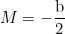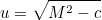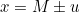OSDev.orghttps://forum.osdev.org/ Poh-Shen Loh’s new method to solving quadratic equationhttps://forum.osdev.org/viewtopic.php?f=11&t=55940 Page 1 of 1

 Author: Klakap [ Mon Oct 18, 2021 12:04 pm ] Post subject: Poh-Shen Loh’s new method to solving quadratic equation Maybe you already heard something about this(it is more than one year old!), but if not, this may be useful for you. This new method make calculating of quadratic equations much much easier than before.1. Edit equation to algebraic form2. Key to success is that a=1 Fortunately if not, you just simple divide whole equation3. Now calculate M:4. Now calculate u:5. And results are your!That’s all! No ugly big formula!Detailed explanation from author why it works and how he found it, is on https://www.poshenloh.com/quadraticdetail/I hope this will help you.Author: Octocontrabass [ Mon Oct 18, 2021 12:59 pm ] Post subject: Re: Poh-Shen Loh’s new method to solving quadratic equation Well, let's work this backwards a bit. Start by substituting the equation for u into the equation for x:Hm. That's starting to look a bit familiar. What happens if we substitute the equation for M into the equation for x too?A bit of algebra and you'll have the quadratic formula. (At least when a=1.)I'm not sure I'd call this a new method, but it is a good demonstration of how a difficult problem can be broken down into several easier ones.

 Author: nullplan [ Mon Oct 18, 2021 1:02 pm ] Post subject: Re: Poh-Shen Loh’s new method to solving quadratic equation Klakap wrote:That’s all! No ugly big formula!I beg to differ. This method is known in Germany (in only slightly different form) as the midnight formula (reason being, you should be able to recite it even if awoken at midnight with no warning). I've used this way to solve quadratics all my life, certainly before 2019. And I never understood the fascination I've seen in others with the general formula. You see, in my book of formulas, there was apparently a typo in the general formula, and it was too complicated for my taste, anyway, so I just always used the normal formula.No, if you want a novel way without a formula, you can use al-Khwarizmi's original method. Bring the equation into the form x² + px = q. Geometrically, the left hand side is an area, so the right hand side must be an area as well. In particular, the left hand side is a square of unknown side length plus a rectangle with one known and one unknown side, and that all adds up to a known area. But we can rearrange the left hand side: If we bisect the rectangle along the side p, then we get two rectangles with side lengths x and p/2. We can paste these onto the unknown square on the right and the bottom (this will go easier if you make a picture, but I have no scanner handy and my paint skills suck). Then the resulting figure is almost a square. In fact, we would only need to add a square with side length p/2 to complete a square, and we can add to the left hand side if we also add the same to the right hand side. Then on the left we have a square with side length x + p/2, and on the right we have a known area quantity: (x + p/2)² = q + p²/4. All that is left to do is to take the root and subtract the p/2 to isolate the x. And of course, in order to get both solutions, you have to put a +- in front of the square root: x = -p/2 +- sqrt(q + p²/4).

 Author: Klakap [ Tue Oct 19, 2021 1:16 am ] Post subject: Re: Poh-Shen Loh’s new method to solving quadratic equation Occontrabass wrote:I'm not sure I'd call this a new method, but it is a good demonstration of how a difficult problem can be broken down into several easier ones.Yes, I also think this should not be called new method, new thing here is explanation how we got this. It is more intuitive than other explanations(watch video). But for me, this final three-line solution is easier to remember than whole formula.nullplan wrote:No, if you want a novel way without a formula, you can use al-Khwarizmi's original method.That’s interesting thing. I did not now about this.

 Page 1 of 1 All times are UTC - 6 hours Powered by phpBB © 2000, 2002, 2005, 2007 phpBB Grouphttp://www.phpbb.com/